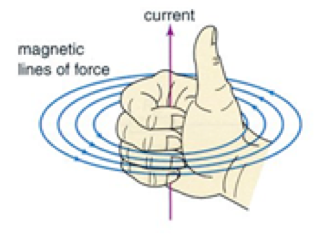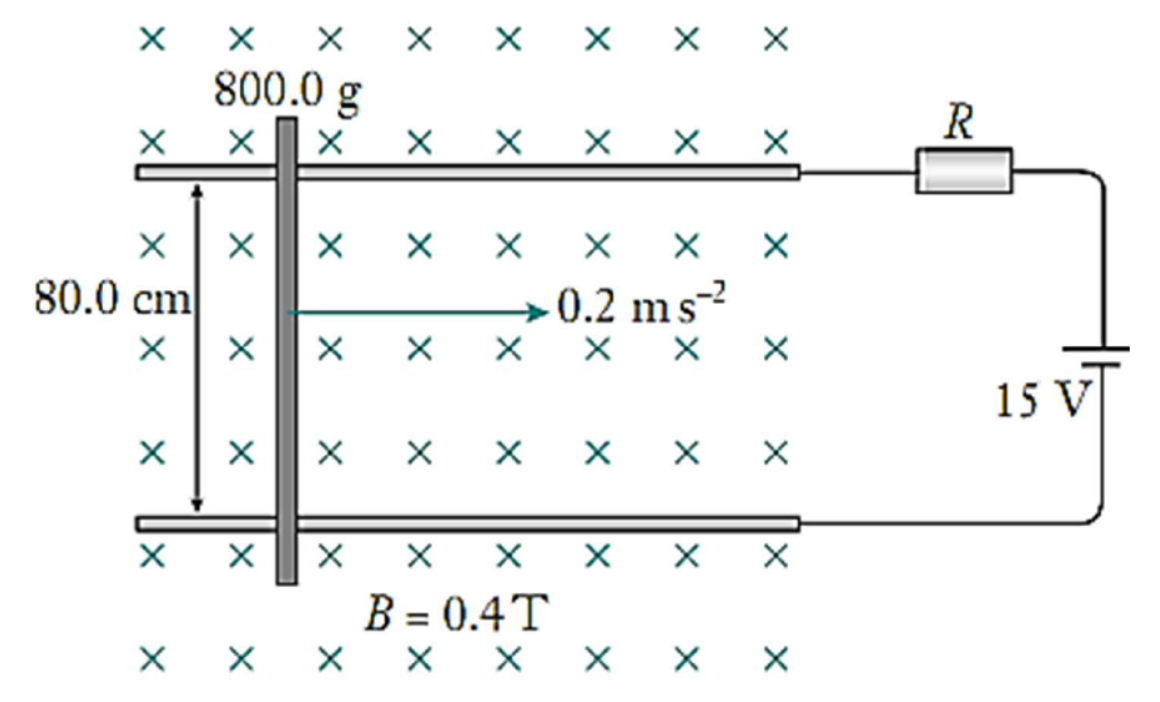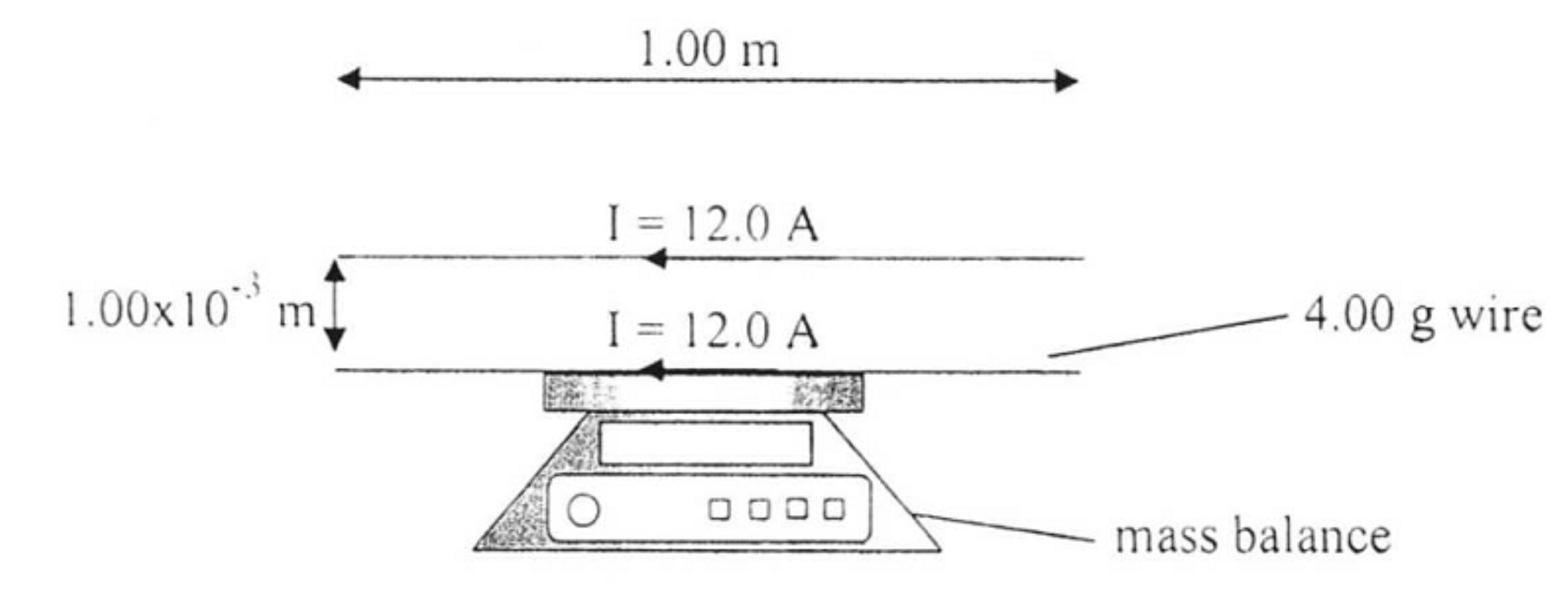# Interaction Between Two Parallel Current-carrying Wires

This is part of the HSC Physics course under the topic The Motor Effect

### HSC Physics Syllabus

• conduct a quantitative investigation to demonstrate the interaction between current-carrying wires
• analyse the interaction between two parallel current-carrying wires F/l=\mu_0/(2\pi) (I_1 I_2)/r and determine the relationship between the International System of Units (SI) definition of an ampere and Newton's Third Law of Motion.

### Force Between Two Parallel Current-carrying Conductors

This video will quantitatively investigate the interaction between parallel current-carrying wires. It will introduce the equation used to calculate the force generated.

### Why is a Force Generated?

A current carrying conductor generates its own magnetic field. The orientation of this magnetic field can be determined by using the right-hand ruleTwo current carrying conductors produce magnetic fields which interact.

• Magnetic fields whose field lines travel in the same rotational direction lead to an attractive interaction.
• Magnetic fields whose field lines travel in the opposite rotational direction lead to a repulsive interaction

This is why the orientation of the currents and thus magnetic fields of the two parallel conductors determines whether they repel or attract.

• parallel conductors with the same direction of current attract
• parallel conductors with the opposition direction of current repel

### Quantitative Investigation of Interaction Between Two Parallel Conductors

The magnitude and direction of the force between two parallel conductors can can be investigated by resting one of the two conductors on an electronic balance. The second conductor is fixed directly above the conductor on the scale. Changes in scale reading will indicate the magnitude and direction of force.

• Increase in mass reading means a repulsive force and currents are travelling in opposite directions
• Decrease in mass reading means an attractive force and currents are travelling in the same direction

### SI Definition of Ampere

The SI definition of an ampere is:

'A constant current magnitude which when flowing two parallel conductors of infinite length, of negligible cross-sectional area and placed 1 m apart, would produce a force of 2xx10^(-7) N/m between these conductors.'

Newton's third law is linked to this definition since the same magnitude of force is experienced by both conductors, but in opposite directions.

Practice Question 1

A 12.0 cm long wire is carrying a current of 3.0 A at 30° to a magnetic field of strength 0.9 T. Determine the magnetic magnitude and direction of the force on the wire. (2 marks)

Practice Question 2

Two conducting rails of negligible resistance are placed 80.0 cm apart. A conducting rod also with negligible resistance is placed across the rails. The mass of the rod is 800.0 g and there is negligible friction between the rod and rails. A 15.0 V battery is connected to the rails through a resistor. It was noticed that when a magnetic field of strength 0.4 T was applied at right angles to the inclined rails, the rod was sliding along the frictionless rails at a constant acceleration of 0.2 ms-2.(a) Calculate the net force acting on the rod (1 mark)

(b) Calculate the current in the circuit (2 marks)

(c) Calculate the resistance of the resistor (1 mark)

Practice Question 3

A wire of length 1.00 m and of mass 4.00 g is placed on top of a laboratory mass balance, which is calibrated to read in grams. A second wire, also 1.00 m long, is placed  m above is so that the two wires are parallel. A current of 12.0 A flows through each wire, in the direction shown in the diagram below.(a) Calculate the force acting on the two parallel conductors. (2 marks)

(b) Calculate the reading on the mass balance. (2 marks)

﻿Previous section: ﻿The Motor Effect

Next section: Electromagnetic Induction